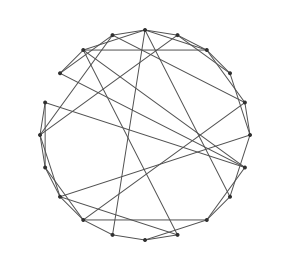Small-world graph model generator

This generator creates small-world graphs of arbitrary size.

This model generates a ring of n nodes where each node is connected to its k nearest neighbors in the ring (k/2 on each side, which means k must be even). Then it process each node of the ring in order following the ring, and “rewiring” each of their edges toward the not yet processed nodes with randomly chosen nodes with a probability beta.

Usage

You must provide values for n, k and beta at construction time. You must ensure that k is event, that n » k » log(n) » 1. Furthermore, beta being a probability it must be between 0 and 1.

By default, the generator will produce a placement for nodes using the `xyz` attribute.

This generator will produce the ring of nodes once `begin()` has been called. Then calling `nextEvents()` will rewire one node at a time return true until each node is processed, in which case it returns false. You must then call `end()`.

Example

Here is an example of use:Reference

This generator is based on the Watts-Strogatz model.

• Collective dynamics of ‘small-world’ networks,
• Watts, D.J. and Strogatz, S.H.,
• Nature 393 (6684): pp 409–10,
• doi:10.1038/30918,
• PMID 9623998. 1998.